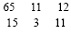# User Forum

Subject :IMO    Class : Class 9

In the given question, two rows of numbers are given. The resultant number in each row is to be worked out separately based on the following rules and the question below the rows is to be answered. The operations of numbers progress from left to right.
Rules :
(i) If an even number is followed by another even number they are to be added.
(ii) If an even number is followed by a prime number they are to be multiplied.
(iii) If an odd number is followed by an even number, the even number is to be subtracted from the odd number.
(iv) If an odd number is followed by another odd number, the first number is to be added to the square of the second number.
(v) If an even number is followed by a composite odd number, the even number is to be divided by the odd number.What is the sum of the resultants of the rows?
(a) 366
(b) 66
(c) 264
(d) 462